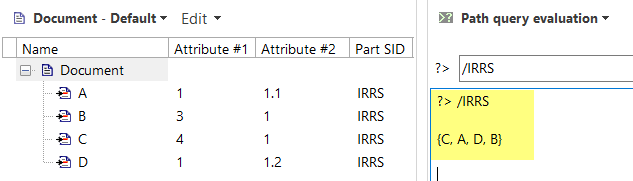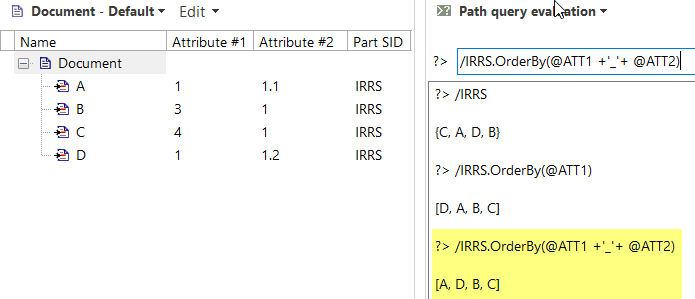Start a new topic

## How to double sort by two different attributes in Path Language

Often it is needed to do sort in two steps, based on two different  attributes. Today there is no support in the SystemWeaver Path Language to do that. One can think of using OrderBy twice, but that will not do the job. Because, applying the second OrderBy will overwrite the first one.

However, it is possible to do double sorting using + Operator and OrderBy, where:

• + Operator performs an addition operation of the two operands (i.e., attribute values).
• OrderBy returns a list with the objects of the set in order according to the given expression.

Example:

Consider the following structure, which is a document with sections where a section can has two attributes (Attribute #1[SID = ATT1] and Attribute #2 [SID = ATT2]).If there is a need to sort by an attribute value, i.e., Attribute #1, then OrderBy can be used. See below.When a duple sort is needed, i.e., order by Attribute#1 and Attribute#2, that can be done by combining the values of the two attributes and then sorting based on the result. See below.Where the result of combining the values of the two attributes ( ATT1 and ATT2) is:The same pattern of combining attributes and then order by the result can be applied for a combination of Part_Attribute and Item_Attribute. For example, consider the following structureWhere the result of combining the Part_Attribute[SID = ATT3] and Item_Attribute[SID = ATT2] is:Login to post a comment